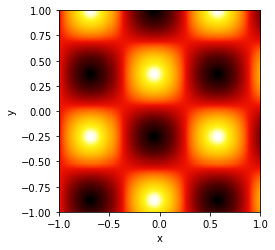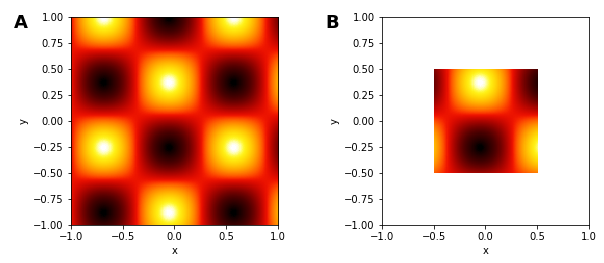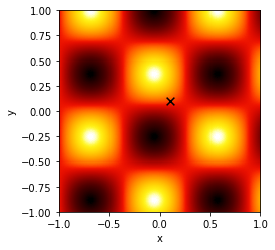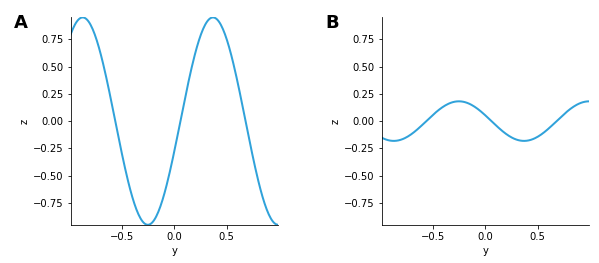# Image¶

Title
Image Element
Dependencies
Matplotlib
Backends
Matplotlib
Bokeh
In :
import numpy as np
import holoviews as hv
hv.extension('matplotlib')Image represents a regularly sampled 2D grid of an underlying continuous space of intensity values, which will be colormapped on plotting. The grid of intensity values may be specified as a NxM sized array of values along with a bounds, but it may also be defined through explicit and regularly spaced x/y-coordinate arrays of shape M and N respectively. The two most basic supported constructors of an Image therefore include:

Image((X, Y, Z))



where X is a 1D array of shape M, Y is a 1D array of shape N and Z is a 2D array of shape NxM, or equivalently:

Image(Z, bounds=(x0, y0, x1, y1))



where Z is a 2D array of shape NxM defining the intensity values and the bounds define the (left, bottom, right, top) edges of four corners of the grid. Other gridded formats which support declaring of explicit x/y-coordinate arrays such as xarray are also supported. See the Gridded Datasets user guide for all the other accepted data formats.

In :
ls = np.linspace(0, 10, 200)
xx, yy = np.meshgrid(ls, ls)

bounds=(-1,-1,1,1)   # Coordinate system: (left, bottom, right, top)
img = hv.Image(np.sin(xx)*np.cos(yy), bounds=bounds)
img

Out:Slicing, sampling, etc. on an Image all operate in this continuous space, whereas the corresponding operations on a Raster work on the raw array coordinates.

In :
img + img[-0.5:0.5, -0.5:0.5]

Out:Notice how, because our declared coordinate system is continuous, we can slice with any floating-point value we choose. The appropriate range of the samples in the input numpy array will always be displayed, whether or not there are samples at those specific floating-point values. This also allows us to index by a floating value, since the Image is defined as a continuous space it will snap to the closest coordinate, to inspect the closest coordinate we can use the closest method:

In :
closest = img.closest((0.1,0.1))
points = hv.Points([closest])

print('The value at position %s is %s' % (closest, img[0.1, 0.1]))

img * points.opts(color='black', marker='x', s=60)

The value at position (0.105, 0.095) is 0.12934720170231848

Out:We can also easily take cross-sections of the Image by using the sample method or collapse a dimension using the reduce method:

In :
img.sample(x=0) + img.reduce(x=np.mean)

Out:The constructor of Image attempts to validate the input data by ensuring it is regularly sampled. In some cases, your data may be not be regularly sampled to a sufficiently high precision in which case you qill see an exception recommending the use of QuadMesh instead. If you see this message and are sure that the Image element is appropriate, you can set the rtol value in the constructor to allow a higher deviation in sample spacing than the default of 10e-6. Alternatively, you can set this globally using hv.config.image_rtol as described in the Installing and Configuring user guide.

One additional way to create Image objects is via the separate ImaGen library, which creates parameterized streams of images for experiments, simulations, or machine-learning applications.

For full documentation and the available style and plot options, use hv.help(hv.Image).